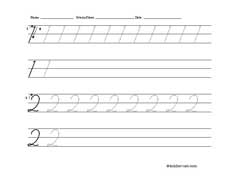Cursive Tracing Cursive Writing/Tracing Cursive Writing Cursive Numbers
HOME
ARTS AND CRAFTS
GAMES
WORKSHEETS
 Letters Numbers Shapes Cursive Preschool Letters Flash cards Names
BIRTHDAY IDEAS
SPORTS
TRAVEL
COMMENTS
LINKS
 Follow Toddler-net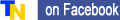# Cursive Numbers Worksheets.

This cursive number worksheets were designed for kids to learn and practice their handwriting skills. Each worksheet contains two rows of two numbers each for tracing and/or writing.
There are three sets of cursive number worksheets below: one is for tracing, second has a row of numbers for tracing and a row for writing. The third set has two rows of two numbers each for writing.
Clicking on the worksheet image below will open worksheet detail page, from which you can download printable worksheet in PDF format.

## 1. CURSIVE NUMBER TRACING WORKSHEETS

Cursive numbers 1 and 2 tracing worksheet
Cursive numbers 3 and 4 tracing worksheet
Cursive numbers 5 and 6 tracing worksheet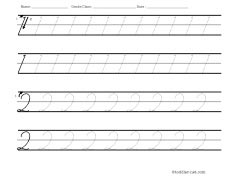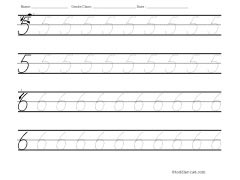Cursive numbers 7 and 8 tracing worksheet
Cursive numbers 9 and 0 tracing worksheet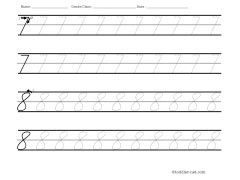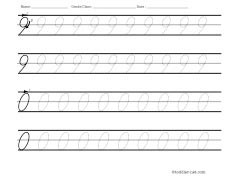## 2. CURSIVE NUMBER WORKSHEETS FOR TRACING AND WRITING

Cursive numbers 1 and 2 tracing and writing worksheet
Cursive numbers 3 and 4 tracing and writing worksheet
Cursive numbers 5 and 6 tracing and writing worksheet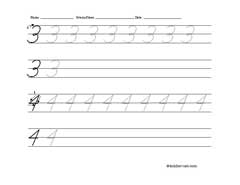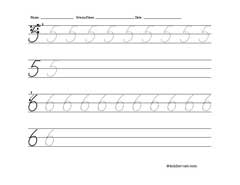Cursive numbers 7 and 8 tracing and writing worksheet
Cursive numbers 9 and 0 tracing and writing worksheet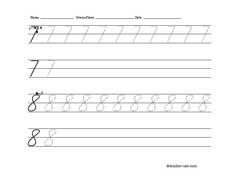## 3. CURSIVE NUMBER WRITING WORKSHEETS

Cursive numbers 1 and 2 writing worksheet
Cursive numbers 3 and 4 writing worksheet
Cursive numbers 5 and 6 writing worksheet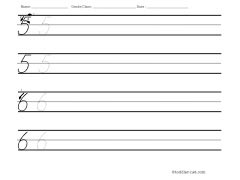Cursive numbers 7 and 8 writing worksheet
Cursive numbers 9 and 0 writing worksheet
Blank writing page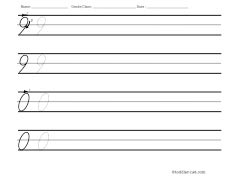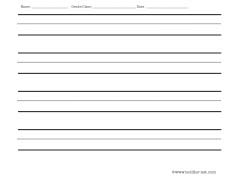Other similar pages:
Number worksheets for preschoolers will help younger children to learn numbers.As a first step in learning cursive handwriting we suggest: Tracing worksheets for cursive letters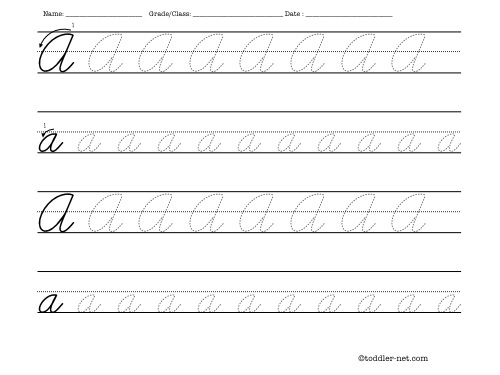Children may trace cursive letters and practice handwriting using our combined worksheets for tracing and writing cursive letter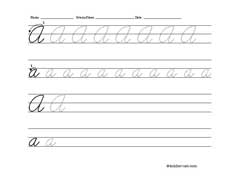Copyright 2009 Toddler-net.com
webmaster@toddler-net.com